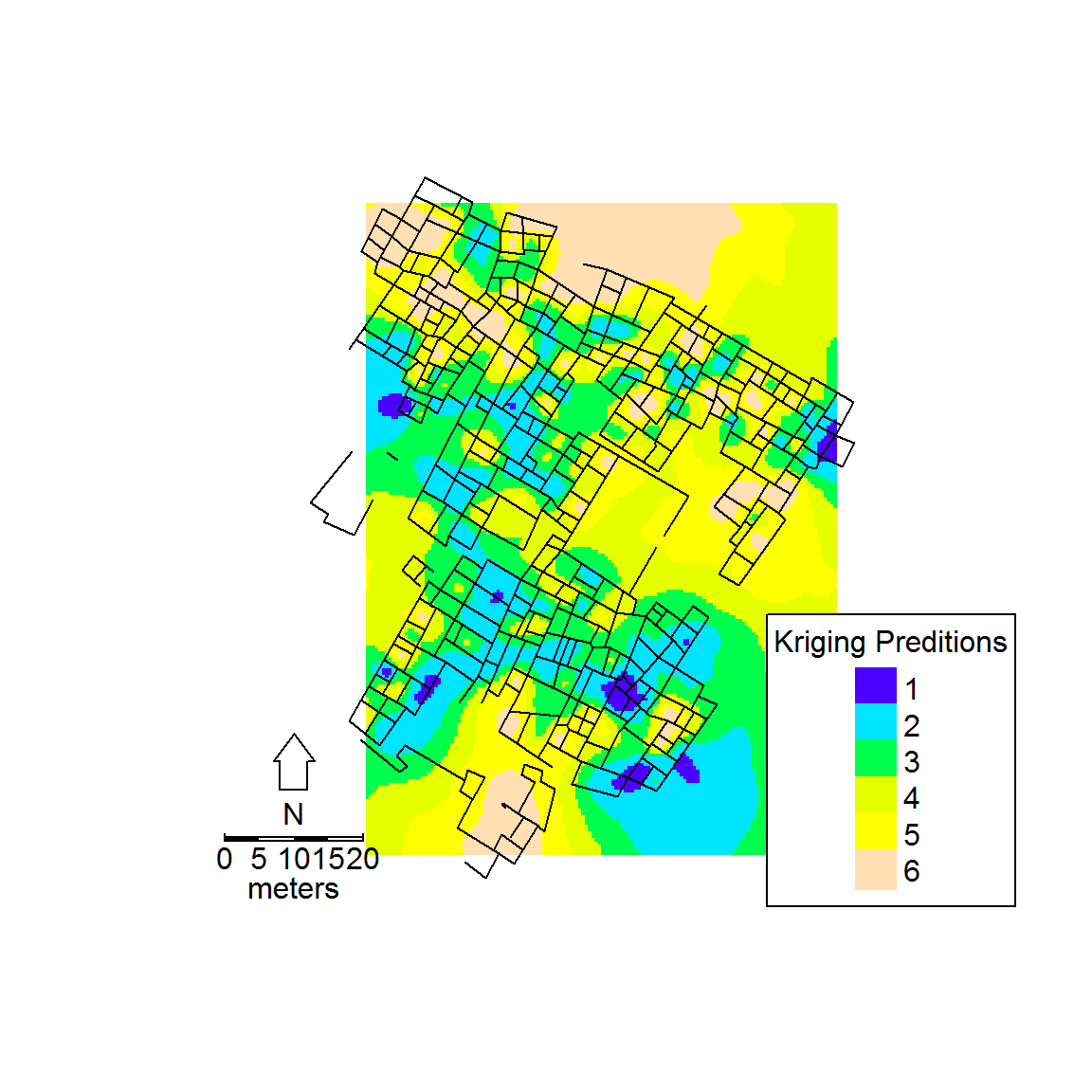The purpose of this document is to help walk you through using map data in R for your first problem set assignment. For this problem set, you start with a shapefile containing the outlines of rooms at Turkey Creek pueblo (from your Lowell 1988 reading) and a *.csv file containing information on 250 excavated rooms at that site including the room number, the X and Y coordinates of the centroid of those rooms (rounded for simplicity), the height in terms of the average number of stone courses in the walls, the area of the room, and the weight of ceramics recovered from those rooms.The first assignment asks you to use R to calculate new variables based on what you’ve been given and then create and interpret maps based on these data.

The first step is simply getting the data in to R. In order to import a shapefile we’re going to use the “maptools” package and the “readShapeLines” command to read in a linear shape file. We could also use “readShapePoly” or “readShapePoints” to read in polygon or point shapefiles (see “maptools” documentation for more info). Following this we will also read in the Turkey.csv file containing information on specific rooms.

library(maptools)

turkey.s <- readShapeLines("Turkey")  #read in Turkey.shp (note that you do not need the .shp)
turkey <- read.csv(file = "Turkey.csv", header = T)  # read in csv file

Now we’ll plot the shapefile we just imported and then add red points representing the centroid of each room for which we have data.

plot(turkey.s)  #plot shapefile
# Note that the next two lines do exactly the same thing. In the first example we
# use the argument turkey[,2:3] as the columns containing the X and Y coordinates
# are the second and third column in turkey. In the second we use turkey$EAST and # turkey$NORTH to call those same columns. In both examples the argument pch=16
# just refers to the R indicator for a filled circle, col='red' makes the points
# red, and cex=0.5 means that the points will be plotted at 0.5 times the default
# size. The 'points' command works like the 'plot' command but simply adds points
# to an existing plot rather than starting a new plot.
points(turkey[, 2:3], pch = 16, col = "red", cex = 0.5)
points(turkey$EAST, turkey$NORTH, pch = 16, col = "red", cex = 0.5)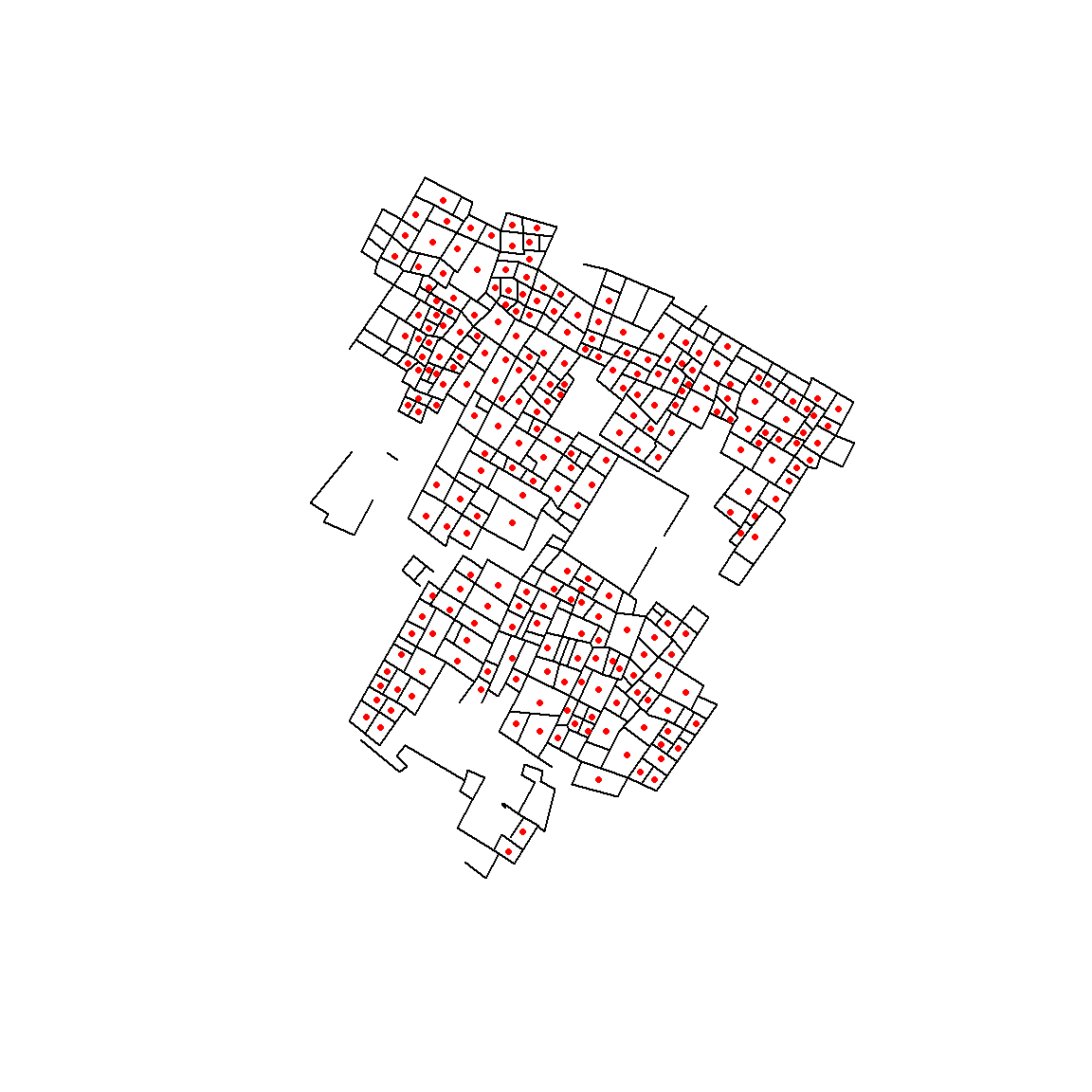Now let’s take a look at the room data we imported using the “head” command.

head(turkey)  # show the first few lines of turkey
##   ROOM EAST NORTH HEIGHT AREA WEIGHT
## 1  221   54     7    2.5  7.8   27.1
## 2  218   58    13    2.8  2.8    6.8
## 3  203   96    28    3.5  5.8    8.6
## 4  204   80    28    2.5 25.1   13.7
## 5  205   92    30    3.8  4.5    0.8
## 6  209   98    34    4.0  9.8    3.1

As specified in your assignment, I want you to explore the relationship between the density of ceramic materials found in each room and the average height of walls (in terms of number of courses). The first thing we need to do then is calculate a column for density and add it to our data.

We calculate density here simply as the weight of ceramics in relation to the estimated volume of each room. Since we don’t have measurements of how much fill was removed from each room, we’ll use the height of walls as a proxy. Thus density = weight / (area * height). Let’s calculate a new column below and then round it so that it only includes 1 number past the decimal point for ease of use.

# We're going to create a new column called DENS in turkey containing our density
# calculation
turkey$DENS <- turkey$WEIGHT/(turkey$AREA * turkey$HEIGHT)
# round this column to one place past the decimal (note that it is not necessary
# to include the text 'digits=' and we can just include the 1)
turkey$DENS <- round(turkey$DENS, digits = 1)
# Let's look at the first few rows of turkey again
head(turkey)
##   ROOM EAST NORTH HEIGHT AREA WEIGHT DENS
## 1  221   54     7    2.5  7.8   27.1  1.4
## 2  218   58    13    2.8  2.8    6.8  0.9
## 3  203   96    28    3.5  5.8    8.6  0.4
## 4  204   80    28    2.5 25.1   13.7  0.2
## 5  205   92    30    3.8  4.5    0.8  0.0
## 6  209   98    34    4.0  9.8    3.1  0.1

Now that we’ve created the density variable we want to view the distribution of values we obtained. An easy and quick way to do this is a histogram.

# view histogram of turkey$DENS. The argument breaks indicates how many cells # (bars) to include in the histogram. It is often a good idea to play with this # number. hist(turkey$DENS, breaks = 75, col = "lightblue")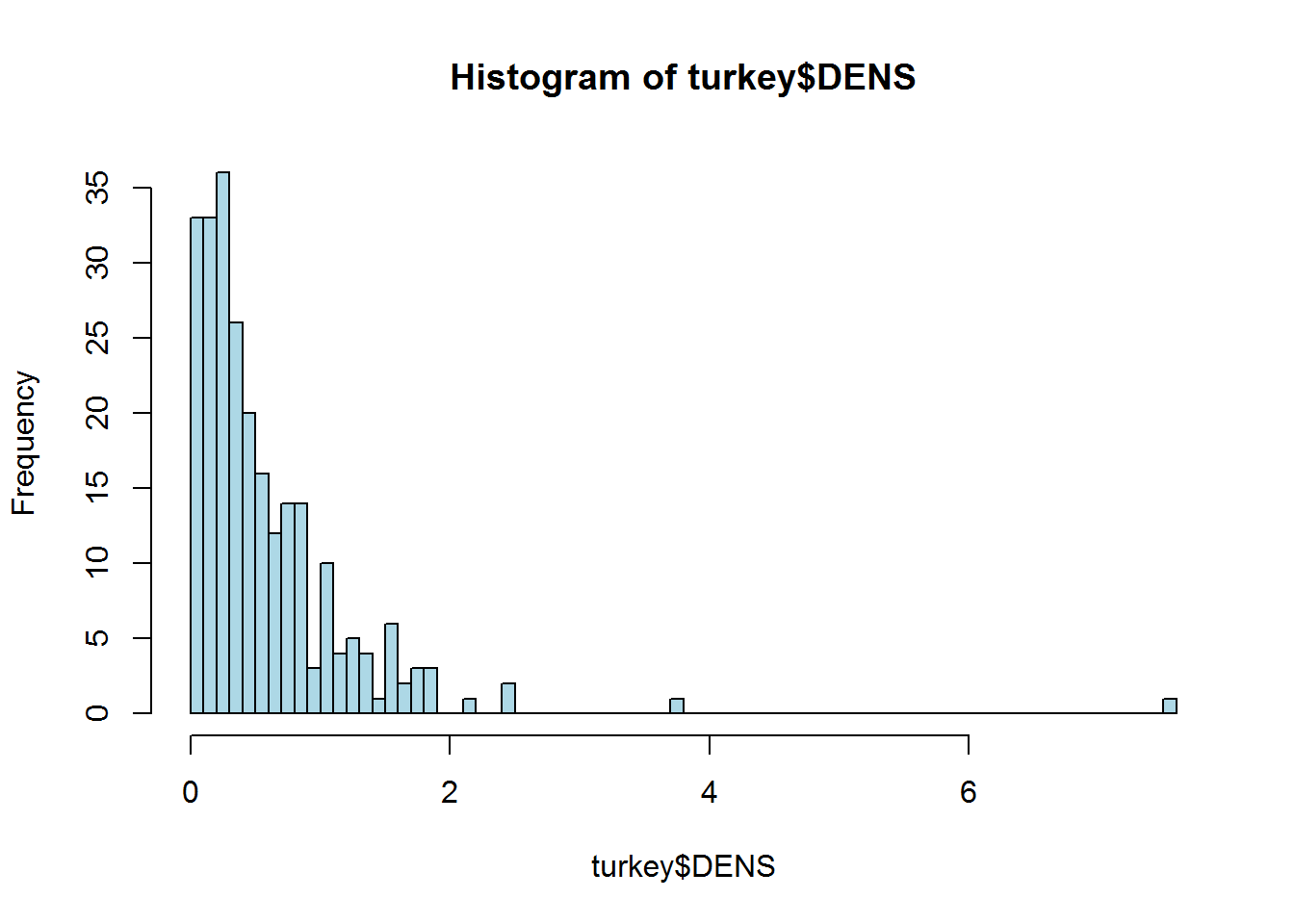As this histogram shows, the distribution of values for DENS is skewed with most values less than 2 but a few in the long tail to the right. For the sake of clarity when displaying these data then it may be a good idea to classify this continuous variable into a number of discrete categories (ranges). There are a number of ways to do this in R and we’ll use the “cut” command. For this command we must select a number of cut offs/breakpoints for our groups and then create a new variable containing those new group assignments. For this example, I will give you the cut offs to use (Selected after viewing the histogram and a bit of experimentation). We will define 6 groups here and designate them 0 through 5 using the cut points at 0, 0.1-0.2, 0.3-0.4, 0.5-0.6, 0.7-0.8, and 0.9 and greater. We will call this new column in turkey DENSGRP.

# the cut command needs you to 'bracket' each group including the upper and lower
# bounds for each group. Since we want our first group to include rooms with only
# 0 for density we indicate this by providing two values for the range from -Inf
# to 0.1 (excluding values of exactly 0.1 because we used the right=F argument).
# The next group will then include values of 0.1-0.3, 0.3-0.5 and so on. We
# provide a value of Inf (infinity) at the end to let the cut command know to
# group everything greater than 0.9 in the same group. The argument 'right=F'
# simply tells the cut comman that the intervals should be closed on the left
# (see ?cut for more details).
temp <- cut(turkey$DENS, c(-Inf, 0.1, 0.3, 0.5, 0.7, 0.9, Inf), right = F) # let's look at a few values to see what this returns head(temp) ##  [0.9, Inf) [0.9, Inf) [0.3,0.5) [0.1,0.3) [-Inf,0.1) [0.1,0.3) ## 6 Levels: [-Inf,0.1) [0.1,0.3) [0.3,0.5) [0.5,0.7) ... [0.9, Inf) This isn’t exactly what we want as the ranges provided by the cut command aren’t that easy to work with. We can simply coerce this into a numeric field to make a new variable ranging from 1-6. # this command takes the intervals from the cut command and coverts them to # sequential numbers. turkey$DENSGRP <- as.numeric(temp)
# let's look at turkey again
head(turkey)
##   ROOM EAST NORTH HEIGHT AREA WEIGHT DENS DENSGRP
## 1  221   54     7    2.5  7.8   27.1  1.4       6
## 2  218   58    13    2.8  2.8    6.8  0.9       6
## 3  203   96    28    3.5  5.8    8.6  0.4       3
## 4  204   80    28    2.5 25.1   13.7  0.2       2
## 5  205   92    30    3.8  4.5    0.8  0.0       1
## 6  209   98    34    4.0  9.8    3.1  0.1       2

Now we’ve got simple numeric assignments for each density category that will be much easier to interpret in plots. Let’s now plot the map of Turkey Creek again with the room centroids, this time with the size of the room centroid determined by the new DENSGRP variable.

plot(turkey.s)
# in the command below we set point size 'cex' to 0.2 times the assignment for
# DENSGRP. The 0.2 simply helps with scaling and avoids points that are too large
# for the scale of our map. You should experiment with differnet multipliers to
points(turkey[, 2:3], pch = 16, col = "red", cex = 0.2 * turkey$DENSGRP)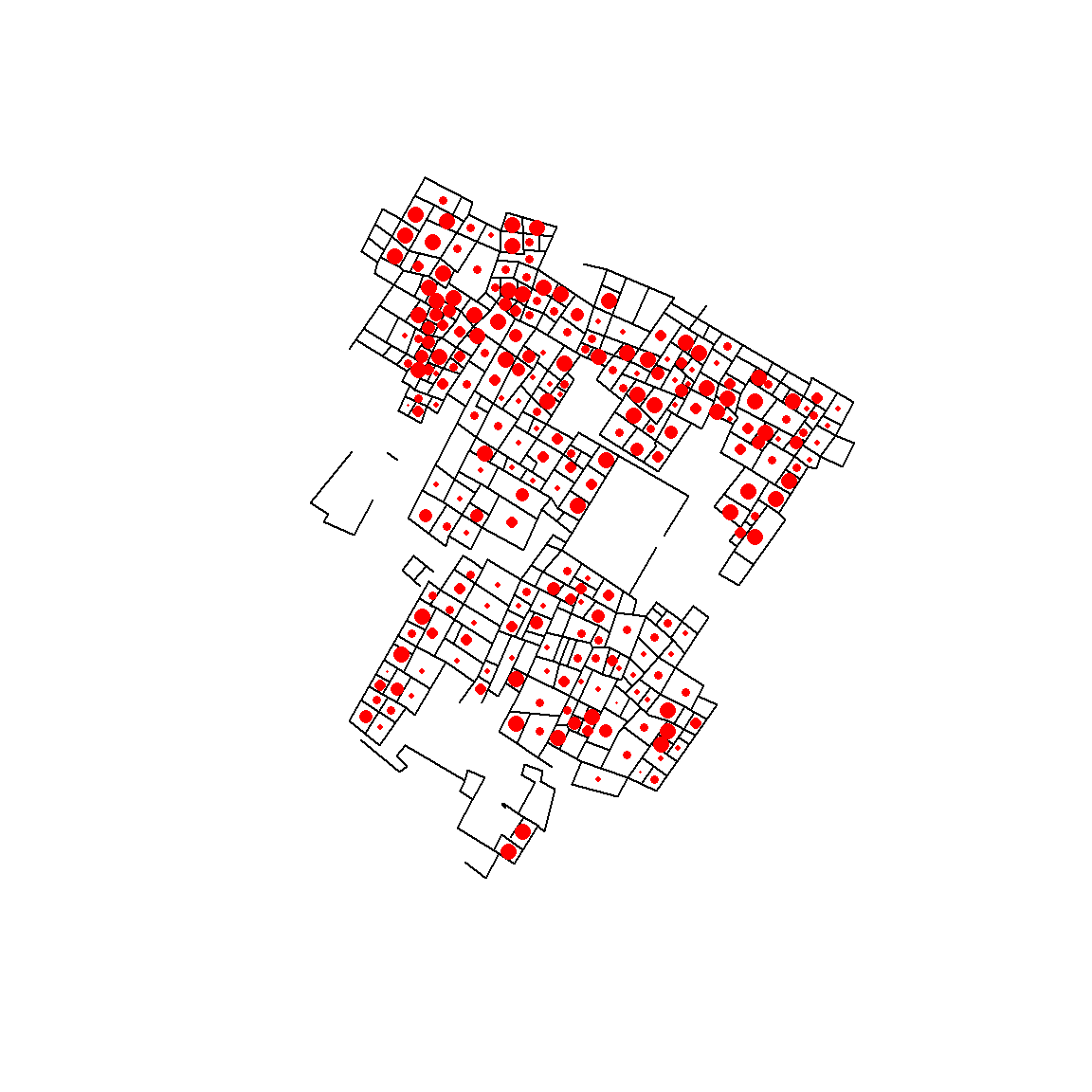The map above helps us see the patterns a bit, but it may also be helpful to use color to differentiate values. I’m going to provide you some useful code below that allows you to create “color ramps” using as many distinct colors as you’d like. You will probably find yourself using this chunk of code or something similar quite a bit. # The following line creates a color scale from yellow to red in 6 steps (the # number of density group values). You can insert any number of colors you choose # in this statement and any number of steps. colscale <- colorRampPalette(c("yellow", "red"))(6) # Now let's plot the map plot(turkey.s) # and now add colors based on the color scale we just created. points(turkey$EAST, turkey$NORTH, pch = 16, cex = 0.2 * turkey$DENSGRP, col = colscale[turkey$DENSGRP])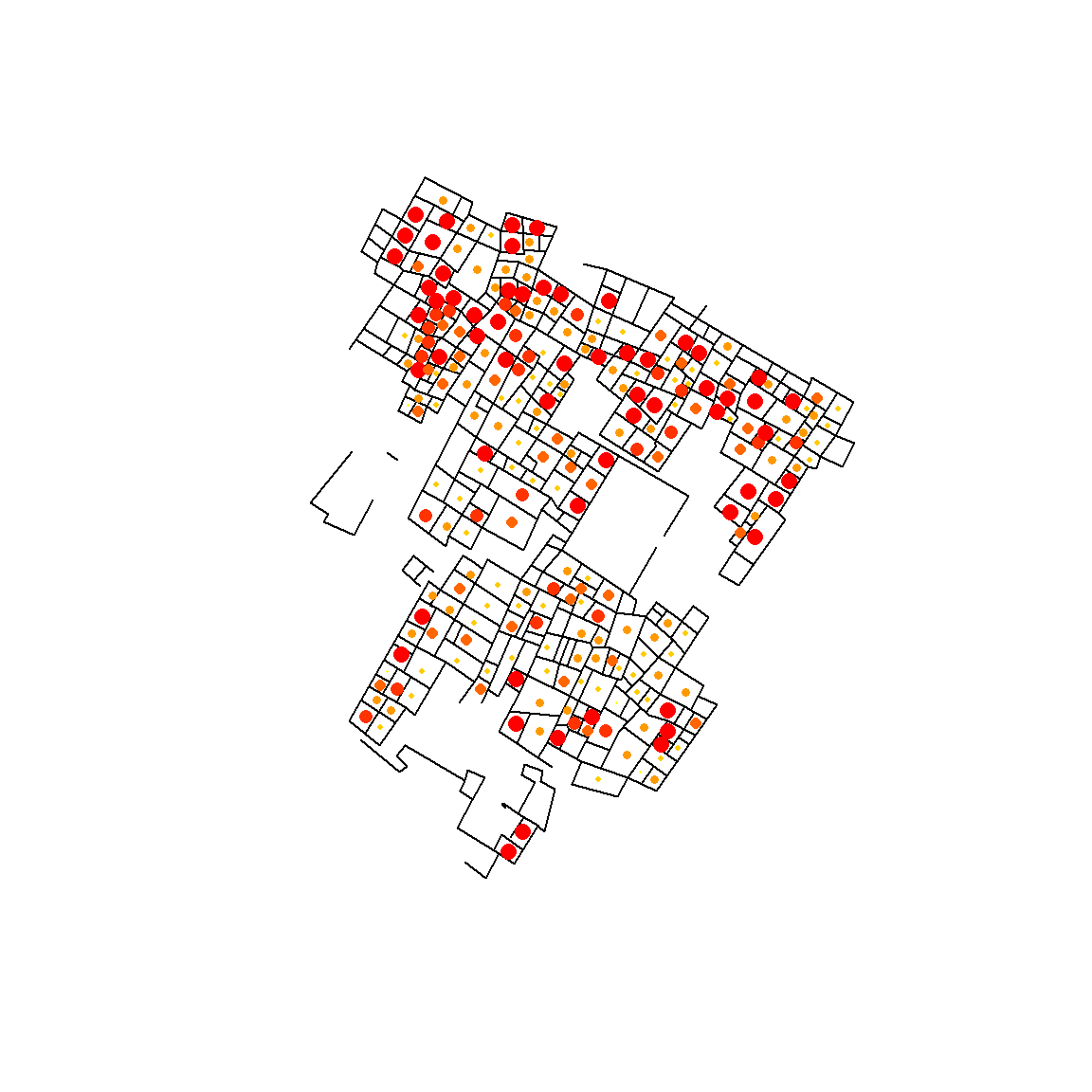That is much more readable but we’re still missing a scale and legend. Let’s add those in. # Set the color scale colscale <- colorRampPalette(c("yellow", "red"))(6) # Plot the map plot(turkey.s) # Add colors based on the color scale we just created. points(turkey$EAST, turkey$NORTH, pch = 16, cex = 0.2 * turkey$DENSGRP, col = colscale[turkey$DENSGRP]) # add a legend in the 'bottomright' of the figure (see ?legend for more options), # with the text indicating values 1-6 [seq(1,6) produces a sequence from 1 to 6]. # pt.cex refers to the size of points in the legend. We use the same 0.2 * # DENSGRP here by using the seq command again. legend("bottomright", legend = seq(1, 6), pch = 16, col = colscale, pt.cex = seq(1, 6) * 0.2, title = "DENSGRP") # Now for a scale. The numbers indicate where (in map units) the center of the # scale should be. In this example I made it 40 map units (meters) long, # indicated that I want 'meters' labeled below the scale, and then gave the scale # 4 divisions which are are 5 meters each (subdiv) library(GISTools) # call package for plotting map scales and north arrows map.scale(-8, 10, len = 40, "meters", ndivs = 4, subdiv = 5) # Now I want to plot a north arrow with the center at the indicated coordinates. # The len argument sets the size of the arrow and lab indicates what I want the # text to say. north.arrow(xb = -8, yb = 25, len = 4, lab = "N")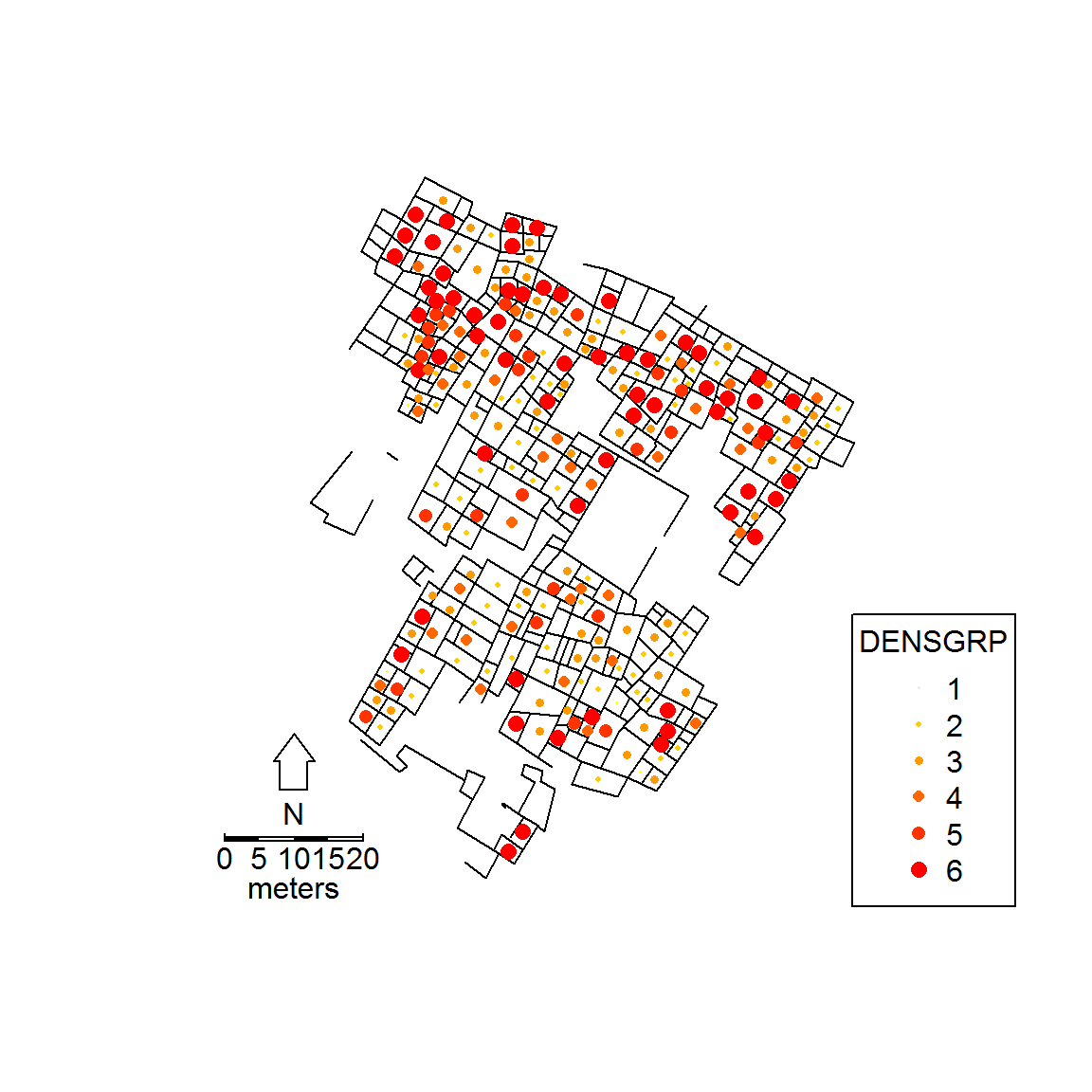Much better but we’re not done yet. Perhaps we also want to map the density of a particular variable using kriging. The easiest way to use this is to simply use the “kriging” package. library(kriging) # The following line creates a kriging density object based on DNSGRP with 200 # pixels in both the x and y axes dens.k <- kriging(turkey$EAST, turkey$NORTH, turkey$DENSGRP, lags = 10, pixels = 200)

# set up plot
plot(turkey.s)
# plot image of kriging density predictions using 'topo colors'
image(dens.k, col = topo.colors(6), add = T)
# Add polyline of Turkey Creek walls
north.arrow(xb = -8, yb = 25, len = 4, lab = "N")